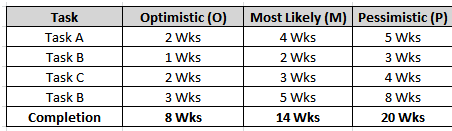# Analysis with the help of PERT and CPM

Program Evaluation and Review Technique (PERT) is a method used to examine the tasked that are in a schedule and determine a variation of the Critical Path Method (CPM). It analyzes the time required to complete each task and its associated dependencies to determine the minimum time to complete a project. It estimates the shortest possible time each activity will take, the most likely length of time, and the longest time that might be taken if the activity takes longer than expected.

The method was developed by the US Navy in 1957 on the Polaris nuclear submarine project.
To conduct PERT Analysis, three time estimates are obtained (optimistic, pessimistic, and most likely) for every activity along the Critical Path. Then use those estimates in the formula below to calculate how much time for each project stage:

Formula: (P+4M+O)/6

• Optimistic Time (O):the minimum possible time required to accomplish a task, assuming everything proceeds better than is normally expected.
• Pessimistic Time (P):the maximum possible time required to accomplish a task, assuming everything goes wrong (excluding major catastrophes).
• Most likely Time (M): the best estimate of the time required to accomplish a task, assuming everything proceeds as normal.Example of the three time estimatesExample of a Critical Path Nodal Diagram

PERT Analysis can be conducted using Microsoft Project.

Critical Path Method

The Critical Path is the longest path of scheduled activities that must be met in order to execute a project.  This is important for Program Managers (PM) to know since any problems that occur on the critical path can prevent a project from moving forward and be delayed.  Earned Value Management (EVM) analysis focuses on the critical path and near critical paths to identify cost and schedule risks. Other schedule paths might have slack time in them to avoid delaying the entire project unlike the critical path. There might be multiple critical paths on a project.The Critical Path is determined when analyze a projects schedule or network logic diagram and uses the Critical Path Method (CPM).  The CPM provides a graphical view of the project, predicts the time required for the project, and shows which activities are critical to maintain the schedule.

The seven (7) steps in the CPM are: 

1. List of all activities required to complete the project (see Work Breakdown Structure (WBS)),
2. Determine the sequence of activities
3. Draw a network diagram
4. Determine the time that each activity will take to completion
5. Determine the dependencies between the activities
6. Determine the critical path
7. Update the network diagram as the project progresses

The CPM calculates the longest path of planned activities to the end of the project, and the earliest and latest that each activity can start and finish without making the project longer. This process determines which activities are “critical” (i.e., on the longest path) and which have “total float” (i.e., can be delayed without making the project longer). 

The CPM is a project modeling technique developed in the late 1950s by Morgan R. Walker of DuPont and James E. Kelley, Jr. of Remington Rand.

## 4 thoughts on “Analysis with the help of PERT and CPM”

error: Content is protected !!